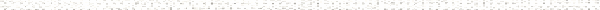A Precalculus Outline

 Chapter 4:  Rational FunctionsObjective: Understanding the concept of asymptoteObjective: Finding vertical asymptotes of a rational functionObjective: Finding horizontal asymptotes of a rational functionObjective: Finding slant/oblique asymptotes of a rational functionObjective: Finding domain algebraicallyObjective: End behaviorObjective: Factoring polynomialsObjective: Simplifying rational expressionsObjective: Polynomial long divisionObjective: Degree of a polynomial functionObjective: Graphing functionsObjective: Finding the Least Common Denominator - Rational ExpressionsObjective: Adding and Subtracting Rational ExpressionsObjective: Graphing Rational FunctionsObjective: Graphing Rational Functions with a Slant / Oblique AsymptoteObjective: Rational functionObjective: Vertical asymptoteObjective: Hole in the graphObjective: Horizontal asymptoteObjective: Slant asymptoteObjective: Sign diagramObjective: Finding domain algebraicallyObjective: Factoring polynomialsObjective: Simplifying rational expressionsObjective: Polynomial long divisionObjective: Degree of a polynomial functionObjective: Graphing functionsObjective: Solve rational equationsObjective: Solve rational equations with extraneous solutionsObjective: Solving a work-rate problemObjective: Solving rational inequalitiesObjective: Sign diagramObjective: Polynomial equationsObjective: Finding a relative maximum of a function graphicallyObjective: Finding a relative minimum of a function graphicallyObjective: Finding a local maximum of a function graphicallyObjective: Finding a local minimum of a function graphicallyObjective: Finding the maximum of a function graphicallyObjective: Finding the absolute maximum of a function graphicallyObjective: Finding the minimum of a function graphically